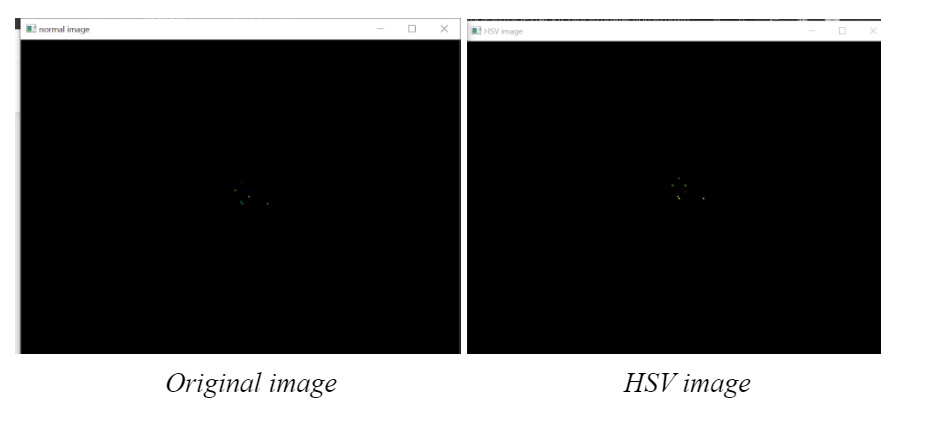top of page
Search

# Determine Color , Contours and Center Using OpenCvIn this article we will show you how to determine the color minor dots, contour and center in an black image background using python 3.7, Open source Computer Vision Library (OpenCV) and numpy.

Advancement of Artificial intelligence computer vision came in the late 1960's. Computer vision should be able to detect the 3d object and 2d pictures. Opencv is a computer vision library. It has been written in the programming languages c and c++. It can be easily run on windows and linux and interface with python language. Computer vision is a field of artificial intelligence that trains computers to interpret and understand the visual world. Color detection using opencv, allows detection of specific color in an image. In this opencv detection system read the image, scan the object, match the color and give the result.if the Color is matched with a defined color pattern by RGB color model then the system gets the correct output as a result.

Image processing is a method to perform some operations on an image, in order to get an enhanced image or to extract some useful information from it. It is a type of signal processing in which input is an image and output may be image or characteristics/features associated with that image.

We are using the jupyter notebook to determine color, contour and center.

Jupyter Notebook IDE: Jupyter Notebook is an open source web-based application which allows you to create and share documents containing live code, equations, visualisations, and narrative text. The IDE also includes data cleaning and transformation, numerical simulation, statistical modelling, data visualisation, and many others.

Step involved in contour and color detection in Python 3.7

Lets begin with a given sample image in either .jpg or .png format and apply object detection in it.

To implement this project the following packages of python 3.7 have to be downloaded and installed.Description of Library used

• Numpy : Numpy is the most basic yet a powerful package for mathematical and scientific computing and data manipulation in python. It is an open source library available in python.

• Cv2: OpenCV is a high performance library for digital image processing and computer vision, which is free and open source.

• Imutils : imutils are a series of convenience functions to make basic image processing functions such as translation, rotation, resizing, skeletonization, and displaying Matplotlib images easier with OpenCV.

Code Snippet :

import cv2
import numpy as np
import imutils

Procedure

Read an image : First a simple image in which processing is to be applied is to be read. It's done using the opencv library. Next convert the image into hsv color model. HSV or Hue Saturation Value is used to separate image luminance from color information. This makes it easier when we are working on or need luminance of the image/frame. HSV is also used in situations where color description plays an integral role. Here, convert the image from RGB to HSV color space and then define a specific range of H-S-V values to detect color

Code Snippet :

img = cv2.imread('data\\left.png')
hsv = cv2.cvtColor(img, cv2.COLOR_BGR2HSV)

Normal image and HSV image :• Now define a Lower color range and upper color range and convert it into numpy array

Code Snippet :

#Red Color
lower_red = np.array([0,100,100])
upper_red = np.array([7,255,255])

# yellow color
lower_yellow = np.array([25,100,100])
upper_yellow = np.array([30,255,255])

#green color
lower_green = np.array([40,70,80])
upper_green = np.array([70,255,255])

#blue color
lower_blue = np.array([90,60,0])
upper_blue = np.array([121,255,255])

#dark teal color
lower_dark_teal = np.array([80,100,100])
upper_dark_teal = np.array([90,255,255])

#dark yellow  color
lower_dark_yellow = np.array([20,100,100])
upper_dark_yellow = np.array([25,255,255])

To perform the actual color detection using OpenCV, see below we use the cv2.inRange function. cv2.inRange function requires three argument images, where we are going to perform a color detection, the second lower range of the color you want to detect, and the third is the upper range you want to detect. Binary mask is returned, where white pixels (255) represent pixels that fall into the upper and lower limit range and black pixels (0) do not.

Code Snippet :

yellow = cv2.inRange(hsv, lower_yellow, upper_yellow)
green = cv2.inRange(hsv, lower_green, upper_green)
blue = cv2.inRange(hsv, lower_blue, upper_blue)
red = cv2.inRange(hsv, lower_red, upper_red)
dark_teal = cv2.inRange(hsv, lower_dark_teal, upper_dark_teal)
dark_yellow = cv2.inRange(hsv, lower_dark_yellow, upper_dark_yellow)

Now we will have to use find Contours to find the number of contours in the Image and find the center of each of them.

cnts1 = cv2.findContours(red,cv2.RETR_TREE,cv2.CHAIN_APPROX_SIMPLE)
cnts1 = imutils.grab_contours(cnts1)

cnts2 = cv2.findContours(yellow,cv2.RETR_TREE,cv2.CHAIN_APPROX_SIMPLE)
cnts2 = imutils.grab_contours(cnts2)

cnts3 = cv2.findContours(blue,cv2.RETR_TREE,cv2.CHAIN_APPROX_SIMPLE)
cnts3 = imutils.grab_contours(cnts3)

cnts4 = cv2.findContours(green,cv2.RETR_TREE,cv2.CHAIN_APPROX_SIMPLE)
cnts4 = imutils.grab_contours(cnts4)

cnts5 = cv2.findContours(dark_teal,cv2.RETR_TREE,cv2.CHAIN_APPROX_SIMPLE)
cnts5 = imutils.grab_contours(cnts5)

cnts6 = cv2.findContours(dark_yellow,cv2.RETR_TREE,cv2.CHAIN_APPROX_SIMPLE)
cnts6 = imutils.grab_contours(cnts6)
• Now Draw the contours for each blob and find the center of blobs using cv2.moments. Image Moment is a particular weighted average of image pixel intensities, with the help of which we can find some specific properties of an image, like radius, area, centroid etc. To find the centroid of the image, we generally convert it into a binary format and then find its center. Using the putText we can display the color name of each blob.

Code Snippet :

for c in cnts1:
cv2.drawContours(img,[c],-1,(0,255,0),3)
# compute the center of the contour
M = cv2.moments(c)
if M["m00"] != 0:
cX = int(M["m10"] / M["m00"])
cY = int(M["m01"] / M["m00"])
else:
cX, cY = 0, 0
cv2.circle(img, (cX, cY), 7, (255, 255, 255), 1)
cv2.putText(img, "red", (cX - 20, cY - 20),cv2.FONT_HERSHEY_SIMPLEX, 2.5, (255, 255, 255), 1)
• Using the following code we can get out

Code Snippet :

cv2.imshow("result",img)
k = cv2.waitKey(0)
if k == 27:
break   

Output :Conclusion :

Computer vision can be used to solve most of the problems with utmost sophistication. We can use both Python and MATLAB for Computer Vision, but we prefer Python because it takes less simulation time than MATLAB. Contours, Centers & colors were detected in the given sample images successfully.

Thanks You,